### IMO Shortlist 2010 problem C6

Kvaliteta:
Avg: 0.0
Težina:
Avg: 8.0
Given a positive integer$k$ and other two integers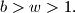$b > w > 1.$ There are two strings of pearls, a string of$b$ black pearls and a string of$w$ white pearls. The length of a string is the number of pearls on it. One cuts these strings in some steps by the following rules. In each step:

(i) The strings are ordered by their lengths in a non-increasing order. If there are some strings of equal lengths, then the white ones precede the black ones. Then$k$ first ones (if they consist of more than one pearl) are chosen; if there are less than$k$ strings longer than 1, then one chooses all of them.
(ii) Next, one cuts each chosen string into two parts differing in length by at most one. (For instance, if there are strings of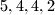$5, 4, 4, 2$ black pearls, strings of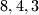$8, 4, 3$ white pearls and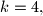$k = 4,$ then the strings of 8 white, 5 black, 4 white and 4 black pearls are cut into the parts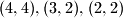$(4,4), (3,2), (2,2)$ and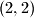$(2,2)$ respectively.) The process stops immediately after the step when a first isolated white pearl appears.

Prove that at this stage, there will still exist a string of at least two black pearls.

Proposed by Bill Sands, Thao Do, Canada
Source: Međunarodna matematička olimpijada, shortlist 2010